# Sort by Last Column in card (heatmap)

I feel like this one is an easy one but I just can't think for the moment. I have a heatmap that shows data over time. I want to sort so that the highest number for the current (or last month of data) is descending from the top. If I do a normal sort it puts the first month in descending order, I just want it to be for the end first.

Also in order to make the months appear in the correct order I sort by the Date field first THEN by the calculated field that is coming up with this number.

Column on far right represents January 2018 and goes back 12 months grouped by month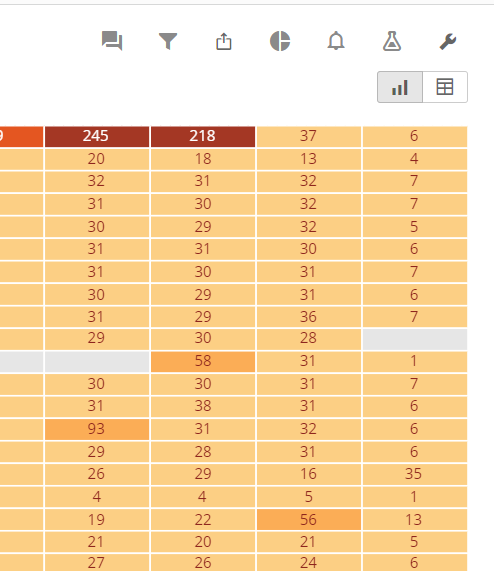• I'm not sure how to get access to redshift (we had access here when I started).  I would contact Domo if you don't have it turned on.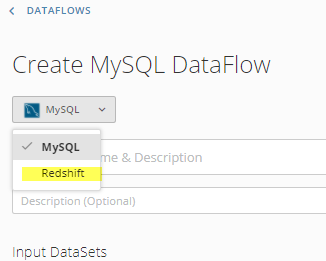Meanwhile, you can accomplish the same thing with a couple of steps inside a MySQL dataflow.

You would first need to create a list of Accounts and the max dates:

SELECT

`Name`

,MAX(`Date`) as `Max Date`

from table_1

GROUP BY `Name`

Then you would join this table to the full data set

SELECT

a.*

,b.`Max Date`

FROM table_1 a

LEFT JOIN table_2 b

ON a.`Name`=b.`Name`

• I would think I about creating a beastmode that would help you sort the data.  Is the last column always the current month?

CASE WHEN `MonthField`=MONTH(CURDATE()) THEN `ValueField` ELSE 0 END

I think you should then be able to sort by this field in descending order first

I'm not really able to test this out without any of the data.  If this doesn't work then could you supply some sample data so I can play around with it some?

• You're kind for trying to help me test Scott.

It didn't work as I had it so I've prepared some test data for you that I made up.

It's the Heatmap card type with the following values:

• Category 1 = Date
• Category 2 = (Rejected % calculated field)
• Series = Name
• Sorting = Date Asc + Rejected % Desc (if I reverse them months sometimes move)
• Grouped by month

The calculation for Rejected % is

• SUM(`Rejected`) / (SUM(Accepted`)+SUM(Rejected))

For this example I only gave once piece of data per month so the SUM doesn't really do anything but it should be the same result.

Based on the test data I gave the goal is that the heatmap goes chronologically from left to right (per column) and that for the last month (always on the far right) the Rejection % be descending order from 100% to 0%.

Does that make sense? Again, thanks for taking the time here

• Is the last month always 12?

I got it to sort correctly this way: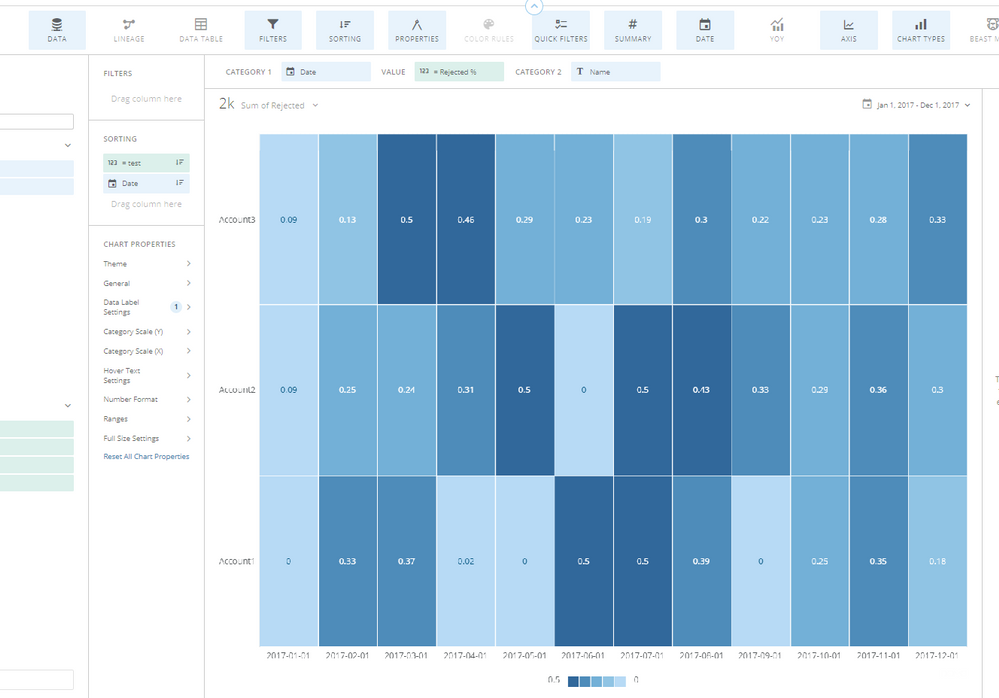The =test beast mode is: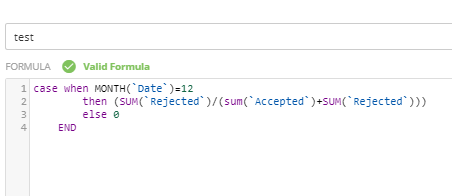Otherwise, if your last month is not always 12 then you would need to add a field in the dataflow that would tell the formula what month to use.  Something like `Max Month` that would be the same value for each line of data and then the beastmode would start with :

CASE WHEN MONTH(`Date`)=`Max Month` THEN...

• OK thanks, I'll have to get back on how/if it worked(Monday). Leaving work shortly. 12 isn't always the last month, in the real one I have it as a rolling 12 months

• Having some trouble figuring which ETL(s) to use to get the max date of the field I have. The calculator can't find a max date, just numerical calculations, date Operations can give me month of date, day of year, etc, but not max date.

• I'm not sure if this is doable via an ETL.  You can accomplish it with a redshift SQL dataflow.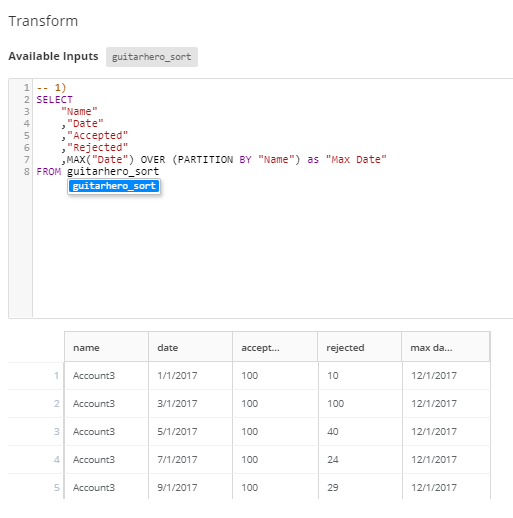Obviously you would want to partition by your unique ID rather than the NAME field, but I didn't have that in my data set.  Let me know if this works for you.  I can try to look into the ETL option, but I'm not sure if it's possible (yet)

• I can look the specific syntax up my I only have access to MySQL DataFlows. Do you know if it has a comparable function? It doesn't like "Partition By". Does Redshift have to be enabled by support?

• Scott,

I think this will do it! Seems to be sorting correctly.  Right now I have a lot of extra stuff going on because we're still iterating on this dataset and it's being pulled in from Excel still and not directly from the database yet which means I have to do ETL to correctly identify NULL fields, field types, etc so all my cards are based on that dataset which is then what this dataset uses as the input so it's Database->Excel->Domo->ETL->MySQL->Dataset. Once we get it connected I should be good with just Database->MySQL->Dataset.

The final beastmode ended up being

CASE WHEN MONTH(`Date`)=MONTH(`Max Date`)
THEN (SUM(`Rejected`) / (SUM(`Accepted`)+SUM(`Rejected`)))
ELSE 0
END

Had to use the MONTH() function on Max Date as well

This discussion has been closed.